Basics of Derivative Pricing and Valuation | IFT World
IFT Notes for Level I CFA® Program
IFT Notes for Level I CFA® Program

# Part 4

## 8. Pricing and Valuation of Options

Let us look at the different types of options.

There are two parties in any option:

• Long party: The buyer of an option or the party that holds the position is the long party.
• Short party: The seller of an option or the party that writes the position is the short party.

Types of options based on purpose:

• Call option: Gives the buyer the right, not the obligation, to buy the underlying asset at a given price on a specified expiration date. The seller of the option has an obligation to sell the underlying asset. The given price is called the strike price or exercise price. For example, if the exercise price is 25, then the buyer has the right to buy the underlying at 25.
• Put option: Gives the buyer the right, not the obligation, to sell the underlying asset at a given price on a specified expiration date. The seller of the option has an obligation to buy the underlying asset.

Types of options based on when they can be exercised:

• European option: This type of option can be exercised only on the expiration date.
• American option: This type of option can be exercised on or any time before the option’s expiration date.

### 8.1 European Option Pricing

Value of European Option at Expiration

Call option

• At expiration, the value of a call option is the greater of zero or the value of the underlying asset minus the exercise price.
•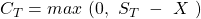Put option

• At expiration, the value of a put option is the greater of zero or the exercise price minus the value of the underlying.
•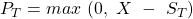Relationship between the value of the option and the value of the underlying

• The value of a European call option is directly related to the value of the underlying.
• The value of a European put option is inversely related to the value of the underlying.

Effect of the Exercise Price

Consider two call options with similar attributes (time to expiration, underlying) but different exercise prices. Assume the exercise price for call option 1 is 25 and that for call option 2 is 30. The right to buy at a lower price of 25 will be more valuable than the right to buy at a higher price of 30.

Similarly, in the case of a put option, the right to sell for a higher price is more valuable than the right to sell for a lower price.

Relationship between the value of the option and the exercise price

• The value of a European call option is inversely related to the exercise price.
• The value of a European put option is directly related to the exercise price.

Moneyness of an option: Indicates whether an option is in, at, or out of the money. The table below shows the moneyness of an option for various relative values of S and X.

 Call Option Put Option In the money S > X S < X At the money S = X S = X Out of the money S < X S > X

Effect of Time to Expiration

Longer-term options should be worth more than shorter-term options. For instance, you have a bought a call option on a stock at an exercise price of $25. Which one would be more valuable to you; an option that expires in a week or an option that expires a year later? Without doubt, the option that expires a year later as it gives enough time for the stock price to increase and make the option valuable. But, is it true for a put option? Partly, yes. The more the time to expiration, the more the opportunity for the stock to fall below its exercise price. What does the put option holder get in return when the underlying falls below the exercise price? Just the exercise price. The longer the holder waits to exercise the option, the lower the present value of the payoff. If the discount rate is high, then a longer time to expiration results in a lower present value for the payoff. Relationship between the value of the option and the time to expiration • The value of a European call option is directly related to the time to expiration. • The value of a European put option can be directly or inversely related to the time to expiration. Inversely related under these conditions: the longer the time to expiration, the deeper the option is in the money, and the higher the risk-free rate. Effect of the Risk-free Rate of Interest If the risk-free rate is high, then the call price is higher. For example, you want to invest in a stock whose price is$100. You can either buy the stock for $100 or buy the call option on the stock for$5. Both these actions give you exposure to the stock. If you buy the call option, then you can invest the remaining \$95. If the interest rates are high, then buying the call option is more valuable because of the interest earned.

In the case of puts, the longer time to expiration with a higher risk-free rate lowers the present value of the exercise price when the option is exercised. That is, when the time to expiration is longer you get the money later and, if the risk-free rate is high, it is discounted by a higher number which lowers the value of the payoff.

Relationship between the value of the option and the risk-free interest rate

• The value of a European call option is directly related to the risk-free interest rate.
• The value of a European put option is inversely related to the risk-free interest rate.

Effect of Volatility of the Underlying

Volatility is good for both call and put options. If the underlying stock becomes more volatile, then the probability of the options expiring deep in the money becomes greater.

Relationship between the value of the option and the volatility of the underlying

• The value of a European call option is directly related to the volatility of the underlying.
• The value of a European put option is directly related to the volatility of the underlying.

Effect of Payments on the Underlying and the Cost of Carry

 Call option Put option Benefits (dividends on stocks, interest on bonds, convenience yield on commodities) Stocks and bonds fall in value when dividends and interest are paid. Decreases because call holders do not get these benefits. Increases Carrying costs Increases as call holders do not incur these costs. Decreases

Relationship between the value of the option and benefits/costs of the underlying

• A European call option is worth less the more benefits that are paid by the underlying. The call option is worth more the more the costs that are incurred in holding the underlying.
• A European put option is worth more the more benefits that are paid by the underlying. The put option is worth less the more costs that are incurred in holding the underlying.

## 9. Lower Limits for Prices of European Options

In this section, we look at the least price one would be interested in paying for a call option. Let us consider a call option on a stock with a strike price of X that expires at time T. The initial price of the underlying at time t = 0 is the underlying price at expiration is and the risk-free rate is r%. If the option is in the money, the payoff at expiration will be S– X. Lowest price of the call option is given by: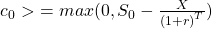Interpreting the above equation:

• It is based on the premise that a call option is equivalent to buying the asset by borrowing the present value of X at a risk-free rate of r%.
• It is the present value of X because you would have to pay X and take delivery of the asset at time T. The payoff for this transaction is S– X (this number can even be negative). In the case of a call option, it can either be zero (out-of-the-money) or ST-X.
• The price of a call option must be at least zero and not a negative value because if it is less than zero, then it cannot be exercised.
•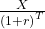is the present value of the exercise price X. So, the lowest price of a call option is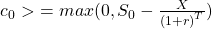Similarly, a put option is equivalent to selling short an asset and investing the proceeds (present value of X) in a risk-free bond at r% for T periods. It will pay X at expiration. A put can never be worth less than zero as the holder cannot be forced to exercise it. The lowest price for a put option is given by: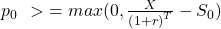TALKING CFA® ← Join a FREE Webinar, Live with Arif Irfanullah on Friday 12th August
This is default text for notification bar